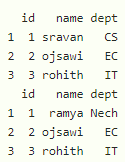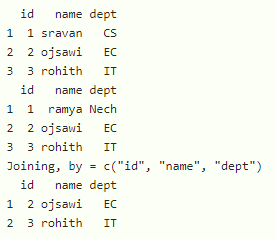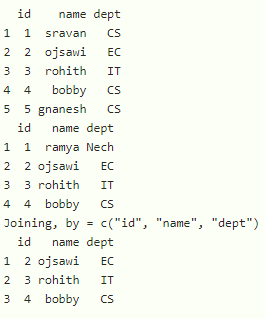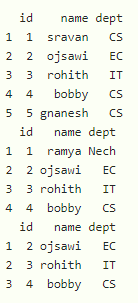# How to find common rows between two dataframe in R?

• Last Updated : 31 Aug, 2021

In this article, we are going to find common rows between two dataframes in R programming language.

For this, we start by creating two dataframes. Dataframes in use:## Method 1: Using inner join

We can get the common rows by performing the inner join on the two dataframes. It is available in dplyr() package

Syntax:

inner_join(data1, data2)

where,

• data1 is the first dataframe
• data2 is the second dataframe

Example: R program to find the common rows in the two dataframes

## R

 `# load the dplyr library``library``(``"dplyr"``)`` ` `# create a dataframe with 3 columns for college1``data1 = ``data.frame``(id=``c``(1, 2, 3),``                   ``name=``c``(``"sravan"``, ``"ojsawi"``, ``"rohith"``),``                   ``dept=``c``(``"CS"``, ``"EC"``, ``"IT"``))`` ` `print``(data1)`` ` `# create a dataframe with 3 columns for college2``data2 = ``data.frame``(id=``c``(1, 2, 3),``                   ``name=``c``(``"ramya"``, ``"ojsawi"``, ``"rohith"``),``                   ``dept=``c``(``"Nech"``, ``"EC"``, ``"IT"``))`` ` `print``(data2)`` ` `# get the common rows by using inner join``inner_join``(data1, data2)`

Output:Example: R program to get the common rows from the dataframes

## R

 `# load the dplyr library``library``(``"dplyr"``)`` ` `# create a dataframe with 3 columns for college1``data1 = ``data.frame``(id=``c``(1, 2, 3, 4, 5),``                   ``name=``c``(``"sravan"``, ``"ojsawi"``, ``"rohith"``, ``"bobby"``, ``"gnanesh"``),``                   ``dept=``c``(``"CS"``, ``"EC"``, ``"IT"``, ``"CS"``, ``"CS"``))`` ` `print``(data1)`` ` `# create a dataframe with 3 columns for college2``data2 = ``data.frame``(id=``c``(1, 2, 3, 4),``                   ``name=``c``(``"ramya"``, ``"ojsawi"``, ``"rohith"``, ``"bobby"``),``                   ``dept=``c``(``"Nech"``, ``"EC"``, ``"IT"``, ``"CS"``))`` ` `print``(data2)`` ` `# get the common rows by using inner join``inner_join``(data1, data2)`

Output:## Method 2 : Using intersect() function

This function is used to get the common rows from the dataframe. This function is available in the generics package so we need to import this package first.

library(“generics”)

Syntax:

generics::intersect(dataframe1, dataframe2)

where

• dataframe1 is the first dataframe
• dataframe2 is the second dataframe

Example: R program to get the common rows by using intersect() function

## R

 `# load the dplyr library``library``(``"dplyr"``)`` ` `# load generics library``library``(``"generics"``)`` ` `# create a dataframe with 3 columns for college1``data1 = ``data.frame``(id=``c``(1, 2, 3, 4, 5),``                   ``name=``c``(``"sravan"``, ``"ojsawi"``, ``"rohith"``, ``"bobby"``, ``"gnanesh"``),``                   ``dept=``c``(``"CS"``, ``"EC"``, ``"IT"``, ``"CS"``, ``"CS"``))`` ` `print``(data1)`` ` `# create a dataframe with 3 columns for college2``data2 = ``data.frame``(id=``c``(1, 2, 3, 4),``                   ``name=``c``(``"ramya"``, ``"ojsawi"``, ``"rohith"``, ``"bobby"``),``                   ``dept=``c``(``"Nech"``, ``"EC"``, ``"IT"``, ``"CS"``))`` ` `print``(data2)`` ` `# get the common rows by using intersect``print``(generics: : ``intersect``(data1, data2))`

Output:My Personal Notes arrow_drop_up